Feeds:
Posts

# Intuitionistic logic

Intuitionistic logic, or constructivist logic, is the symbolic logic system originally developed by Arend Heyting to provide a formal basis for Brouwer‘s programme of intuitionism. The system preserves justification, rather than truth, across transformations yielding derived propositions. From a practical point of view, there is also a strong motivation for using intuitionistic logic, since it has the existence property, making it also suitable for other forms of mathematical constructivism.

##  Syntax

The syntax of formulæ of intuitionistic logic is similar to propositional logic or first-order logic. However, intuitionistic connectives are not interdefinable in the same way as in classical logic, hence their choice matters. In intuitionistic propositional logic it is customary to use →, ∧, ∨, ⊥ as the basic connectives, treating ¬ as the abbreviation ¬A = (A → ⊥). In intuitionistic first-order logic both quantifiers ∃, ∀ are needed.

Many tautologies of classical logic can no longer be proven within intuitionistic logic. Examples include not only the law of excluded middle p ∨ ¬p, but also Peirce’s law ((pq) → p) → p, and even double negation elimination. In classical logic, both p → ¬¬p and also ¬¬pp are theorems. In intuitionistic logic, only the former is a theorem: double negation can be introduced, but it cannot be eliminated.

The observation that many classically valid tautologies are not theorems of intuitionistic logic leads to the idea of weakening the proof theory of classical logic.

###  Sequent calculus

Main article: sequent calculus

Gentzen discovered that a simple restriction of his system LK (his sequent calculus for classical logic) results in a system which is sound and complete with respect to intuitionistic logic. He called this system LJ.

###  Hilbert-style calculus

Intuitionistic logic can be defined using the following Hilbert-style calculus. Compare with the deduction system at Propositional calculus#Alternative calculus.

In propositional logic, the inference rule is modus ponens

• MP: from φ and φ → ψ infer ψ

and the axioms are

• THEN-1: φ → (χ → φ)
• THEN-2: (φ → (χ → ψ)) → ((φ → χ) → (φ → ψ))
• AND-1: φ ∧ χ → φ
• AND-2: φ ∧ χ → χ
• AND-3: φ → (χ → (φ ∧ χ))
• OR-1: φ → φ ∨ χ
• OR-2: χ → φ ∨ χ
• OR-3: (φ → ψ) → ((χ → ψ) → (φ ∨ χ → ψ))
• FALSE: ⊥ → φ

To make this a system of first-order predicate logic, the generalization rules

• ∀-GEN: from ψ → φ infer ψ → (∀x φ), if x is not free in ψ
• ∃-GEN: from φ → ψ infer (∃x φ) → ψ, if x is not free in ψ

are added, along with the axioms

• PRED-1: (∀x φ(x)) → φ(t), if no free occurrence of x in φ is bound by a quantifier quantifying a variable occurring in the term t
• PRED-2: φ(t) → (∃x φ(x)), with the same restriction as for PRED-1

####  Optional connectives

#####  Negation

If one wishes to include a connective ¬ for negation rather than consider it an abbreviation for φ → ⊥, it is enough to add:

• NOT-1′: (φ → ⊥) → ¬φ
• NOT-2′: ¬φ → (φ → ⊥)

There are a number of alternatives available if one wishes to omit the connective ⊥ (false). For example, one may replace the three axioms FALSE, NOT-1′, and NOT-2′ with the two axioms

• NOT-1: (φ → χ) → ((φ → ¬χ) → ¬φ)
• NOT-2: φ → (¬φ → χ)

as at Propositional calculus#Axioms. Alternatives to NOT-1 are (φ → ¬χ) → (χ → ¬φ) or (φ → ¬φ) → ¬φ.

#####  Equivalence

The connective ↔ for equivalence may be treated as an abbreviation, with φ ↔ χ standing for (φ → χ) ∧ (χ → φ). Alternatively, one may add the axioms

• IFF-1: (φ ↔ χ) → (φ → χ)
• IFF-2: (φ ↔ χ) → (χ → φ)
• IFF-3: (φ → χ) → ((χ → φ) → (φ ↔ χ))

IFF-1 and IFF-2 can, if desired, be combined into a single axiom (φ ↔ χ) → ((φ → χ) ∧ (χ → φ)) using conjunction.

####  Relation to classical logic

The system of classical logic is obtained by adding any one of the following axioms:

• φ ∨ ¬φ (Law of the excluded middle. May also be formulated as (φ → χ) → ((¬φ → χ) → χ).)
• ¬¬φ → φ (Double negation elimination)
• ((φ → χ) → φ) → φ (Peirce’s law)

In general, one may take as the extra axiom any classical tautology that is not valid in the two-element Kripke frame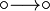(in other words, that is not included in Smetanich’s logic).

Another relationship is given by the Gödel–Gentzen negative translation, which provides an embedding of classical first-order logic into intuitionistic logic: a first-order formula is provable in classical logic if and only if its Gödel–Gentzen translation is provable intuitionistically. Therefore intuitionistic logic can instead be seen as a means of extending classical logic with constructivist semantics.

###  Non-interdefinability of operators

In classical propositional logic, it is possible to take one of conjunction, disjunction, or implication as primitive, and define the other two in terms of it together with negation, such as in Łukasiewicz‘s three axioms of propositional logic. It is even possible to define all four in terms of a sole sufficient operator such as the Peirce arrow (NOR) or Sheffer stroke (NAND). Similarly, in classical first-order logic, one of the quantifiers can be defined in terms of the other and negation.

These are fundamentally consequences of the law of bivalence, which makes all such connectives merely Boolean functions. The law of bivalence does not hold in intuitionistic logic, only the law of non-contradiction. As a result none of the basic connectives can be dispensed with, and the above axioms are all necessary. Most of the classical identities are only theorems of intuitionistic logic in one direction, although some are theorems in both directions. They are as follows:

Conjunction versus disjunction:

•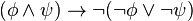•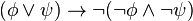•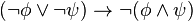•Conjunction versus implication:

••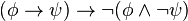••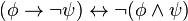Disjunction versus implication:

•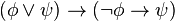••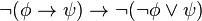•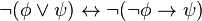Universal versus existential quantification:

•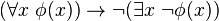•••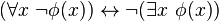So, for example, “a or b” is a stronger statement than “if not a, then b”, whereas these are classically interchangeable. On the other hand, “neither a nor b” is equivalent to “not a, and also not b”.

If we include equivalence in the list of connectives, some of the connectives become definable from others:

•••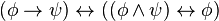•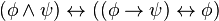•In particular, {∨, ↔, ⊥} and {∨, ↔, ¬} are complete bases of intuitionistic connectives.

As shown by Alexander Kuznetsov, either of the following defined connectives can serve the role of a sole sufficient operator for intuitionistic logic:

••##  Semantics

The semantics are rather more complicated than for the classical case. A model theory can be given by Heyting algebras or, equivalently, by Kripke semantics.

###  Heyting algebra semantics

In classical logic, we often discuss the truth values that a formula can take. The values are usually chosen as the members of a Boolean algebra. The meet and join operations in the Boolean algebra are identified with the ∧ and ∨ logical connectives, so that the value of a formula of the form AB is the meet of the value of A and the value of B in the Boolean algebra. Then we have the useful theorem that a formula is a valid sentence of classical logic if and only if its value is 1 for every valuation—that is, for any assignment of values to its variables.

A corresponding theorem is true for intuitionistic logic, but instead of assigning each formula a value from a Boolean algebra, one uses values from a Heyting algebra, of which Boolean algebras are a special case. A formula is valid in intuitionistic logic if and only if it receives the value of the top element for any valuation on any Heyting algebra.

It can be shown that to recognize valid formulas, it is sufficient to consider a single Heyting algebra whose elements are the open subsets of the real line R. In this algebra, the ∧ and ∨ operations correspond to set intersection and union, and the value assigned to a formula AB is int(ACB), the interior of the union of the value of B and the complement of the value of A. The bottom element is the empty set ∅, and the top element is the entire line R. Negation is as usual defined as ¬A = A → ∅, so the value of ¬A reduces to int(AC), the interior of the complement of the value of A, also known as the exterior of A. With these assignments, intuitionistically valid formulas are precisely those that are assigned the value of the entire line.

For example, the formula ¬(A ∧ ¬A) is valid, because no matter what set X is chosen as the value of the formula A, the value of ¬(A ∧ ¬A) can be shown to be the entire line:

Value(¬(A ∧ ¬A)) =
int((Value(A ∧ ¬A))C) =
int((Value(A) ∩ Value(¬A))C) =
int((X ∩ int((Value(A))C))C) =
int((X ∩ int(XC))C)

A theorem of topology tells us that int(XC) is a subset of XC, so the intersection is empty, leaving:

int(∅C) = int(R) = R

So the valuation of this formula is true, and indeed the formula is valid.

But the law of the excluded middle, A ∨ ¬A, can be shown to be invalid by letting the value of A be {y : y > 0 }. Then the value of ¬A is the interior of {y : y ≤ 0 }, which is {y : y < 0 }, and the value of the formula is the union of {y : y > 0 } and {y : y < 0 }, which is {y : y ≠ 0 }, not the entire line.

The interpretation of any intuitionistically valid formula in the infinite Heyting algebra described above results in the top element, representing true, as the valuation of the formula, regardless of what values from the algebra are assigned to the variables of the formula. Conversely, for every invalid formula, there is an assignment of values to the variables that yields a valuation that differs from the top element. No finite Heyting algebra has both these properties.

###  Kripke semantics

Main article: Kripke semantics

Building upon his work on semantics of modal logic, Saul Kripke created another semantics for intuitionistic logic, known as Kripke semantics or relational semantics .

##  Relation to other logics

Intutionistic logic is related by duality to a paraconsistent logic known as Brazilian, anti-intuitionistic or dual-intuitionistic logic.